In this post, we will see how to calculate Interest under section 234C in Saral Income Tax.

Interest u/s 234C is the interest applied for delayed payment of Advance Tax. This is applied to the shortfall of each due date. For more on computation, details click here.

In Saral Income Tax, to compute Interest u/s 234C, enter the details of income, investments and tax paid in the respective screen. After entering all the details, click on Interest u/s 234 C in the Tax Meter.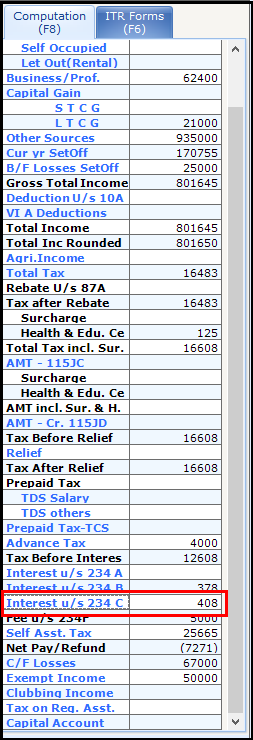You can see the screen of auto computed Interest u/s 234 C.Here, the calculation is explained below:

• The total tax payable is Rs. 12608. Hence, Advance Tax is applicable.
• The first instalment of AT is Rs. 2200 on or before 15th.The total tax paid before 15th Jun is Rs. 4000. Hence, NO shortfall in tax payment and no interest are computed.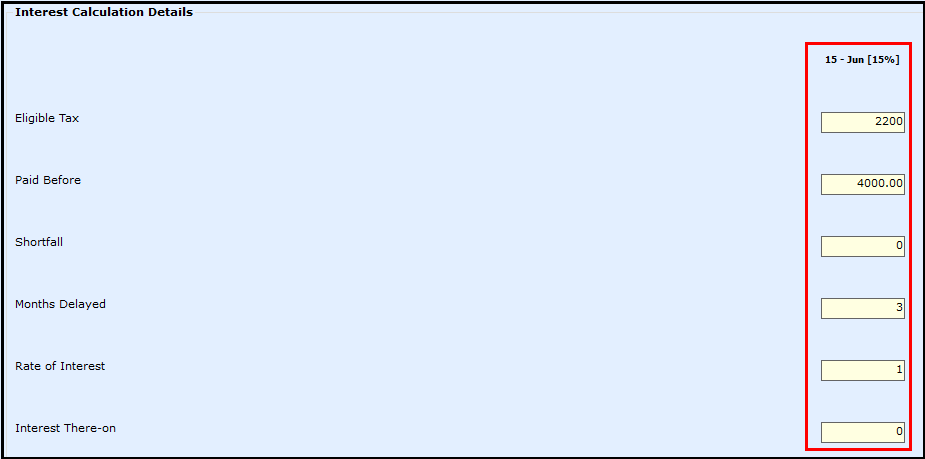• The second instalment payable is Rs. 6599 on or before 15th Whereas, total tax paid before 15thSep is only Rs. 4000. Hence, there is a shortfall of Rs. 2500 (rounded-off) in tax payment and the interest calculated is Rs. 75. i.e. Rs. 2500*1%*3 Months.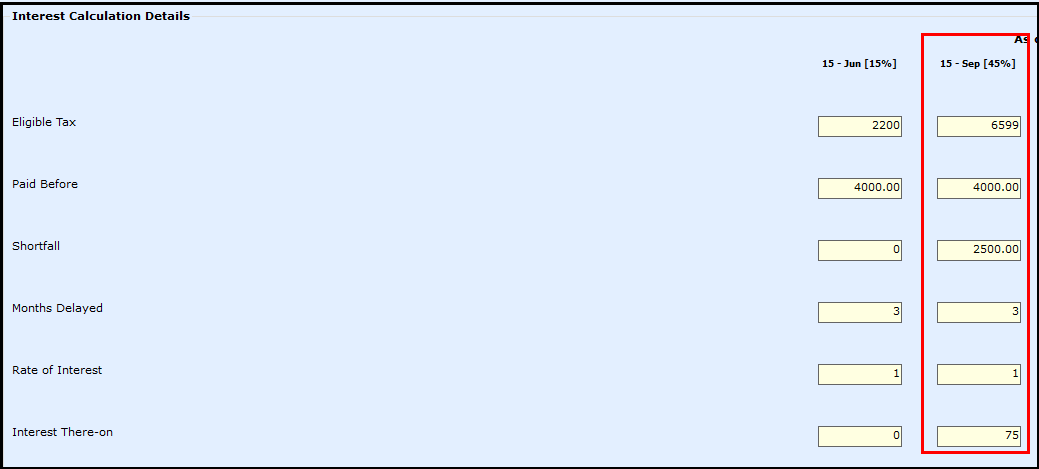Note: The delay of 3 months is calculated from the month of September to December or till the month in which the shortfall is completed paid.

• Similarly, the third instalment payable is Rs. 10998 on or before 15th The total tax paid before 15thDec is only Rs. 4000. Hence, there is a shortfall of Rs. 6900 (rounded-off) in tax payment and the interest calculated is Rs. 207. i.e. Rs. 6900*1%*3 Months.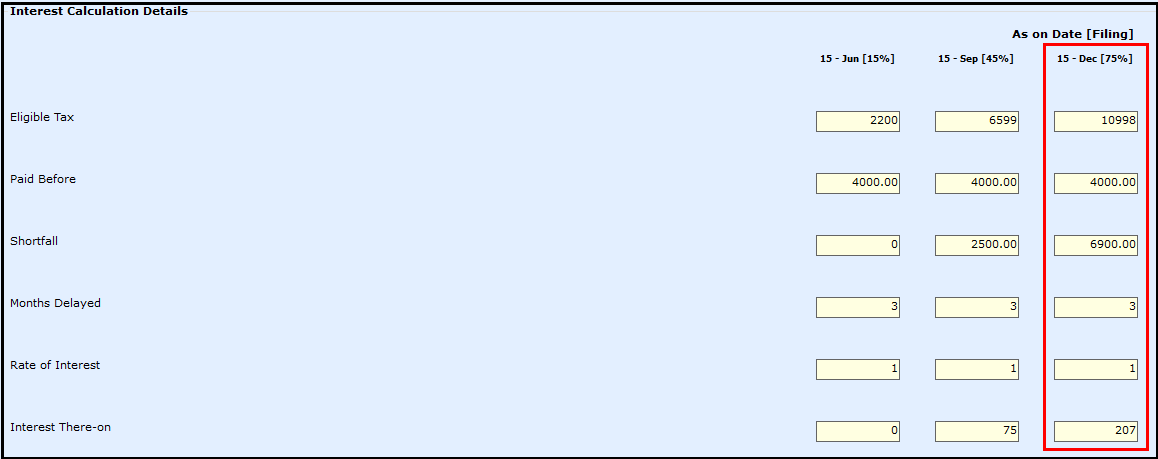• The last instalment payable is Rs. 16600 on or before 15th The total tax paid before 15thMar is only Rs. 4000. Hence, there is a shortfall of Rs. 12600 (rounded-off) in tax payment and the interest calculated is Rs. 126. i.e. Rs. 6900*1%*1 Month.### Note:

The delay of 1 month is calculated from the 15th Mar to 31st Mar.

Lastly, all the interest is put together to consider the Interest u/s 234C which is Rs. 408 in above-illustrated case.

If there are any changes in the value or the user wants to edit the value, then click on Allow Edit and change the value. On click of Save, the value will be added to Net Tax Payable.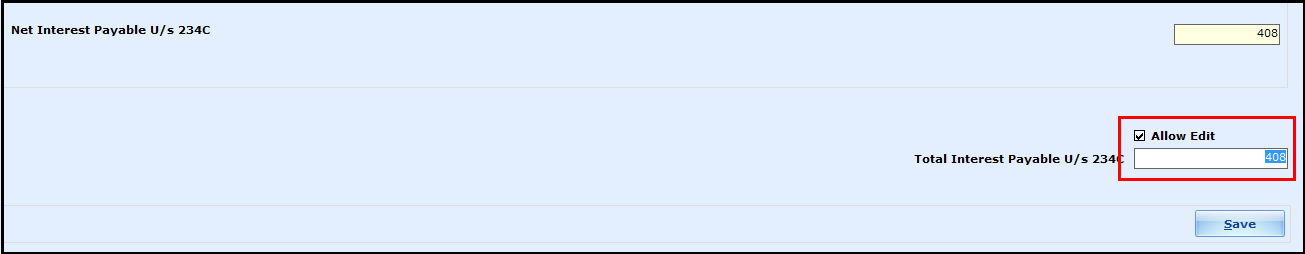This completes the calculation of Interest u/s 234C in Saral Income Tax.